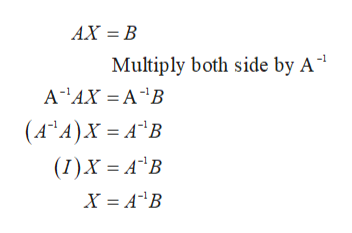# If A, B and X are matrices and AX = B then which of the following statements is correct.Select one:a. X= A-1Bb. X = BAc. X = BA-1d. A = XB

Question
1 views

If A, B and X are matrices and AX = B then which of the following statements is correct.

Select one:
a. X= A-1B
b. X = BA
c. X = BA-1
d. A = XB
check_circle

Step 1

Here we put one condition that A is an invertible matrix otherwise all the option is incorrect.

Let if A is invertible, the multiply AX = B b...help_outlineImage TranscriptioncloseAX B Multiply both side by A |(A A)X A B (I)X = A*B fullscreen

### Want to see the full answer?

See Solution

#### Want to see this answer and more?

Solutions are written by subject experts who are available 24/7. Questions are typically answered within 1 hour.*

See Solution
*Response times may vary by subject and question.
Tagged in

### Math